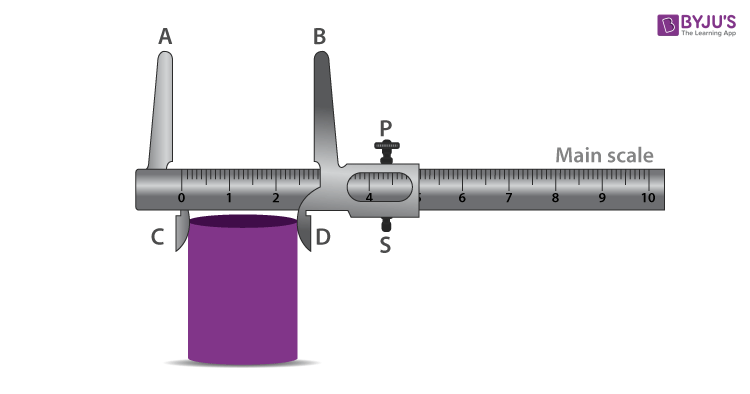# To Measure Diameter of a Small Spherical/ Cylindrical Body Using Vernier’s Callipers

A vernier scale or a vernier calliper is a measuring device that is used to precisely measure linear dimensions. It is a useful tool to measure spherical and cylindrical objects. It has two main scales known as the main scale and the vernier’s scale which are divided into small divisions. Both the scale has two jaws that are perpendicular to the scale. The zeroes of the main scale and vernier scale coincide when the jaws are made to touch each other. The jaws and the metallic strips are designed to measure the diameter of objects. In this experiment, we will learn to measure the diameter of a small spherical/cylindrical body using Vernier Calliper.

## Aim

Use of Vernier’s Calliper to measure the diameter of a small spherical/cylindrical body.

## Apparatus/ Materials Required

• A spherical body such as a pendulum bob or a glass marble
• Vernier’s Caliper
• Magnifying Glass

## Theory

The smallest distance that can be measured along the distance is the least count. It is the difference between one main scale division and one vernier scale division.

$n(V.S.D)=(n-1)M.S.D$

### Formula Used

• Least count of Vernier’s Calliper

• $=\frac{magnitude\,of\,the\,smallest\,divison\,on\,the\,main\,scale}{total\,number\,of\,small\,divisons\,on\,the\,vernier\,scale}$
• Corrected Diameter = Mean Observed DiameterZero Error

## Diagram## Procedure

1. Keep the jaws of the vernier calliper closed. Make sure that the zero of the main scale perfectly coincides with the zero of the vernier scale. If it doesn’t coincide account for the zero error for all observations.
2. Using a magnifying glass, look for the division of the main scale that coincides with the division of the vernier scale. Note down the number of division that coincides with each other. To avoid parallax error, position your eye directly over the division mark.
3. Release the movable jaw by gently loosening the screw. Slide it enough to hold the sphere or the cylindrical body between the jaws AB without any undue pressure. Align the perfectly perpendicular to the diameter of the body. Gently tighten the screw in order to clamp the instrument in this position to the body.
4. Note down the position of the zero of vernier’s scale against the zero of the main scale. Normally, it will not perfectly coincide with any of the divisions on the main scale. Record the main scale division to the left of the zero marks of the vernier scale.
5. Look for the exact coincidence of the vernier scale division with that of the main scale division in the vernier window from the left end to the right. Note down the number N.
6. Multiply the obtained N by the least count of the instrument and add the product to the main scale reading noted in step 4. Make sure to convert the product into proper units for valid addition.
7. Repeat steps 3-6 to get the positions of the body at different positions on its curved surface. Make sure to take three sets of reading in each case.
8. Record the observations in a tabular column with proper reading. If needed, apply zero correction.
9. Find the arithmetic mean of the corrected readings of the diameter of the body.

## Observations

• Least count of Vernier Callipers

I main scale division = 1 mm = 0.1 cm

Number of Venier scale divison N = 10

10 Vernier scale divison = 9 main scale divison

1 Vernier scale divison = 0.9 main scale divison

Vernier Constant = 1 main scale division – 1 vernier scale division

= (1 – 0.9) main scale divisions

= 0.1 main scale divisions

Vernier constant = 0.1 mm = 0.01 cm

• $True\,Reading=Observed\,Reading-(\pm Zero\,error)$
• Table for measuring the diameter of a small/spherical body
 S. No. Main Scale reading, M (cm/mm) Number of coinciding vernier division, N Vernier scale reading, V = N × VC (cm/mm) Measured diameter, M + V (cm/mm) 1 2 3 4
$Zero\,Error=\pm ….cm$

Mean Observed Diameter = ……cm

Corrected Diameter = Mean Observed DiameterZero Error

## Result

The diameter of the given sphere/cylinder is _____ cm.

## Viva Voce

1. What is the principle of a vernier?
2. Answer: The vernier scale uses the alignment of line segments displaced by a small amount to make fine measurements.

3. How is the least count of vernier callipers calculated?
4. Answer: Least count also known as the vernier constant is the difference between one main scale division (1 mm) and one vernier scale division (0.9 mm). It can also be calculated by dividing the smallest unit on the main scale by the total numbers on the vernier scale.

5. Define vernier constant.
6. Answer: Vernier constant is the ratio of the smallest division of the main scale to the number of divisions of the vernier scale.

7. What is parallax error and how can it be avoided?
8. Answer: Parallax is an effect where the direction and position of the object appear to differ when viewed from different lines of sight.

9. What are the precautions to be taken while using Verniers?

Following are the precautions to be taken while using verniers:

• If the vernier scale doesn’t slide smoothly over the main scale, apply grease or machine oil.
• Keep the eye directly over the division mark to avoid parallax error.
• Screw the vernier tightly without undue pressure to avoid damages to the threads of the screw.
11. What are the uses of vernier callipers?